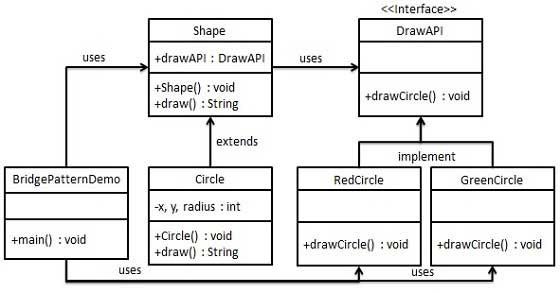# 桥接模式

## 实现### 步骤 1

DrawAPI.java

```public interface DrawAPI {
public void drawCircle(int radius, int x, int y);
}
```

### 步骤 2

RedCircle.java

```public class RedCircle implements DrawAPI {
@Override
public void drawCircle(int radius, int x, int y) {
System.out.println("Drawing Circle[ color: red, radius: "
+ radius +", x: " +x+", "+ y +"]");
}
}
```

GreenCircle.java

```public class GreenCircle implements DrawAPI {
@Override
public void drawCircle(int radius, int x, int y) {
System.out.println("Drawing Circle[ color: green, radius: "
+ radius +", x: " +x+", "+ y +"]");
}
}
```

### 步骤 3

Shape.java

```public abstract class Shape {
protected DrawAPI drawAPI;
protected Shape(DrawAPI drawAPI){
this.drawAPI = drawAPI;
}
public abstract void draw();
}
```

### 步骤 4

Circle.java

```public class Circle extends Shape {

public Circle(int x, int y, int radius, DrawAPI drawAPI) {
super(drawAPI);
this.x = x;
this.y = y;
}

public void draw() {
}
}
```

### 步骤 5

BridgePatternDemo.java

```public class BridgePatternDemo {
public static void main(String[] args) {
Shape redCircle = new Circle(100,100, 10, new RedCircle());
Shape greenCircle = new Circle(100,100, 10, new GreenCircle());

redCircle.draw();
greenCircle.draw();
}
}
```

### 步骤 6

```Drawing Circle[ color: red, radius: 10, x: 100, 100]
Drawing Circle[  color: green, radius: 10, x: 100, 100]
```

App下载